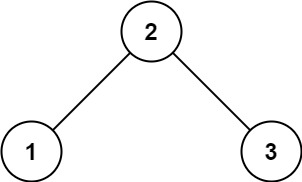# 98. Validate Binary Search Tree / Medium

Given the `root` of a binary tree, determine if it is a valid binary search tree (BST).

A valid BST is defined as follows:

• The left subtree of a node contains only nodes with keys less than the node’s key.
• The right subtree of a node contains only nodes with keys greater than the node’s key.
• Both the left and right subtrees must also be binary search trees.

## Example 1:Input: root = [2,1,3]
Output: true

## Example 2:Input: root = [5,1,4,null,null,3,6]
Output: false
Explanation: The root node’s value is 5 but its right child’s value is 4.

## Constraints:

• The number of nodes in the tree is in the range [1, 10^4].
• -2^31 <= Node.val <= 2^31 - 1

# Solution 1: Recursive

## 效能

### Complexity

• Time Complexity: O(N)
• Space Complexity: O(N)

### LeetCode Result

• Runtime: 12 ms
• Memory Usage: 21.5 MB

# Solution 2: Inorder Traversal is Sorted

## 思路

BST 的特性之一，inorder traversal 的節點值應該會是排序狀態，所以我們將其記錄下來，檢查是否是已排序狀態。

## 效能

### Complexity

• Time Complexity: O(N)
• Space Complexity: O(N)

### LeetCode Result

• Runtime: 13 ms
• Memory Usage: 21.9 MB